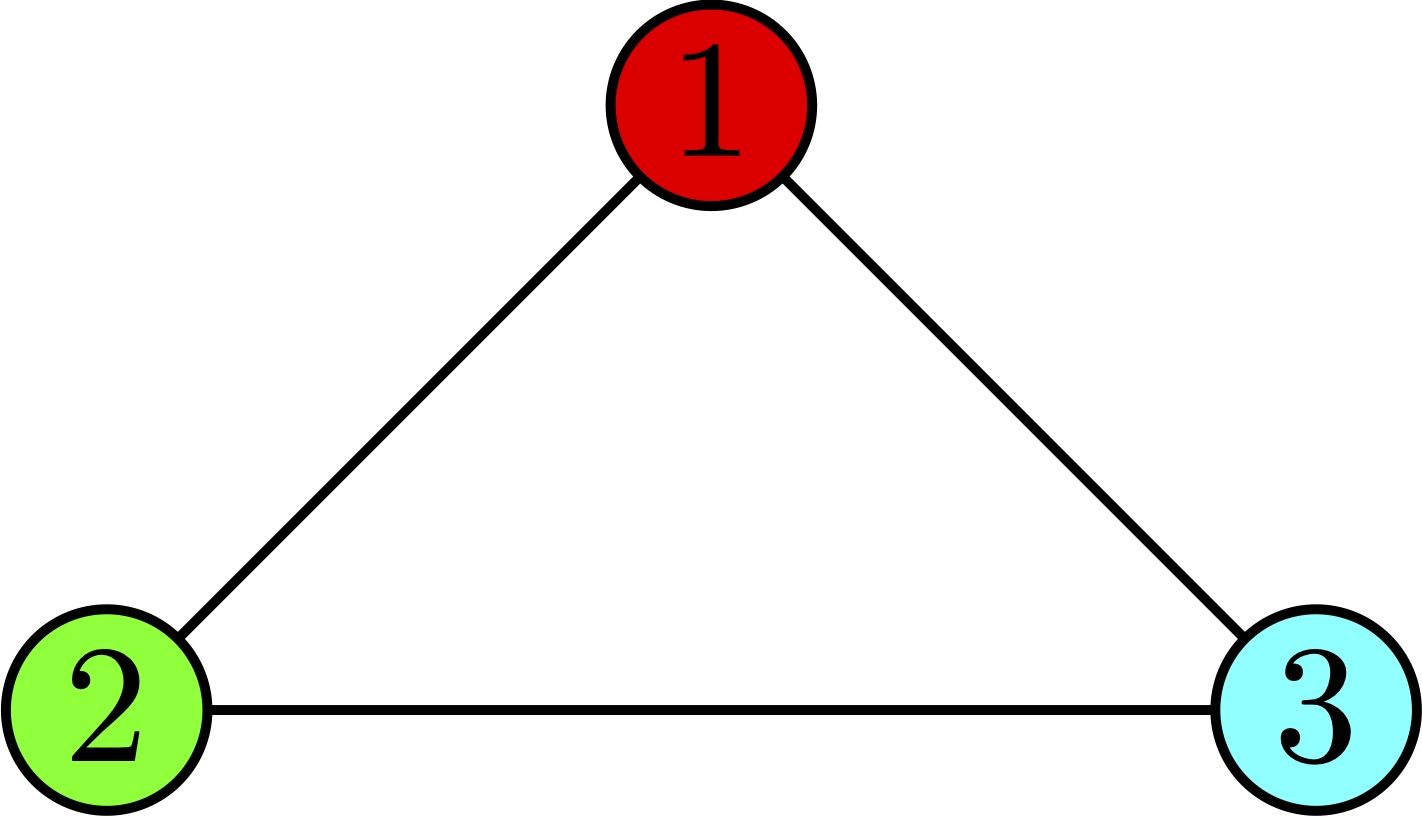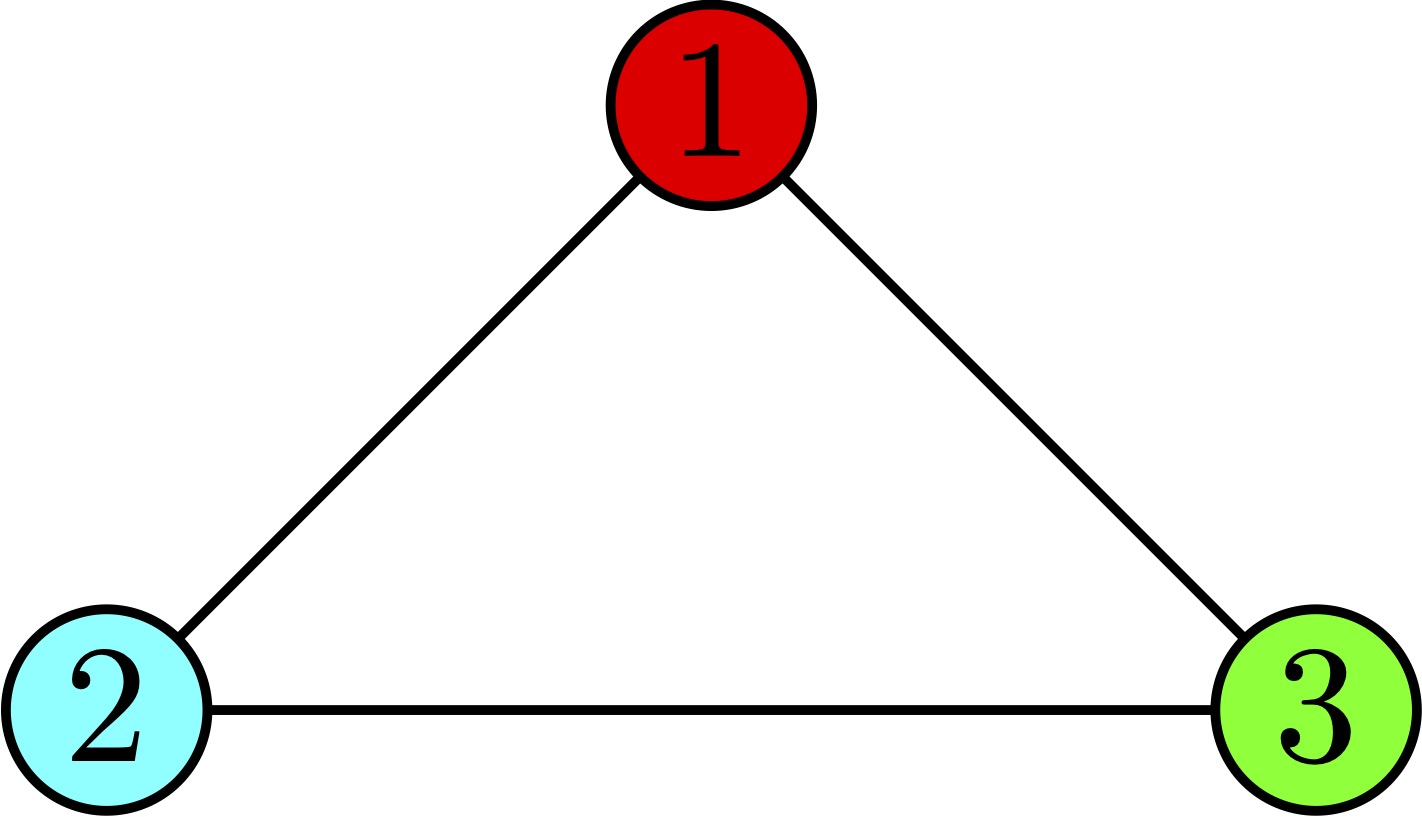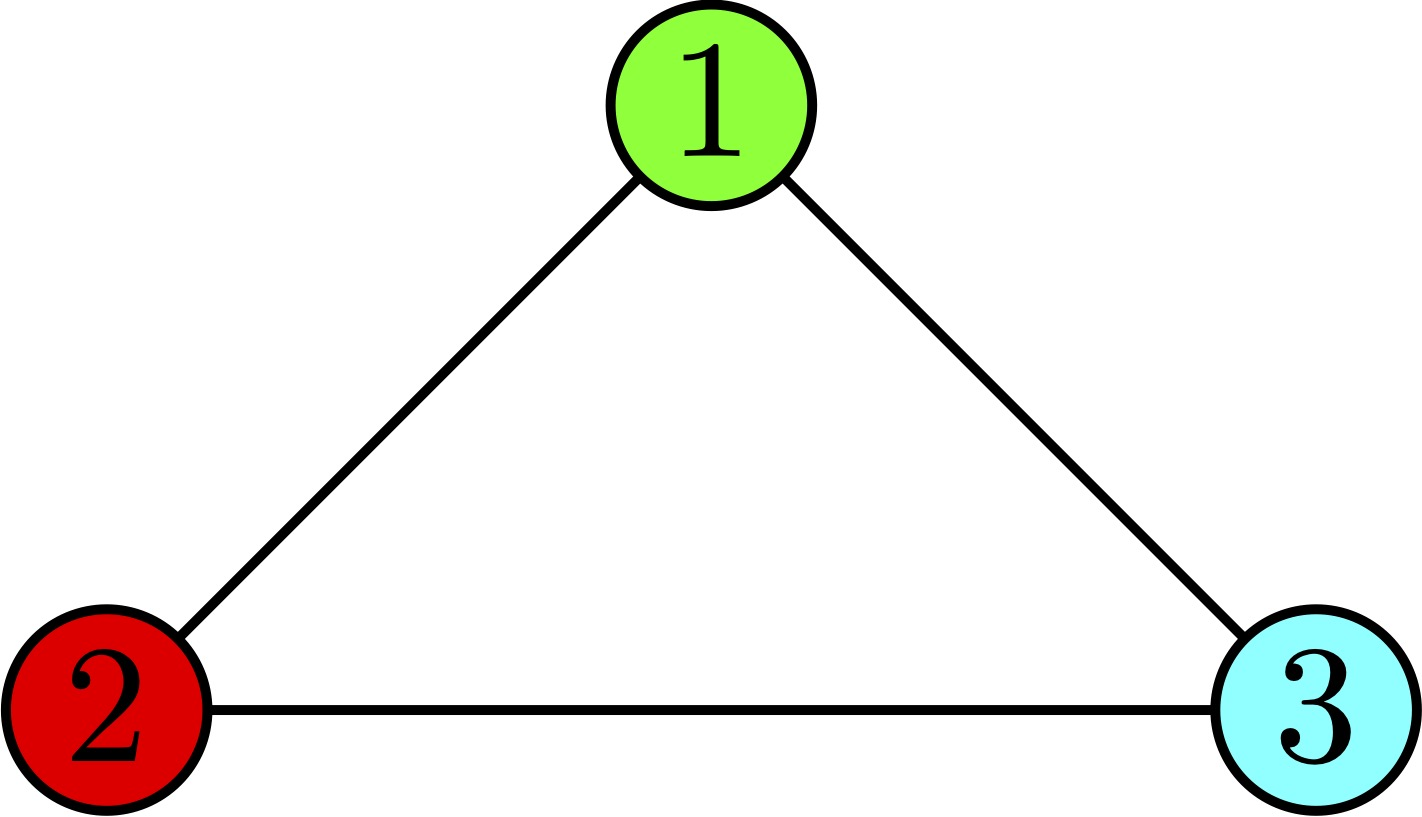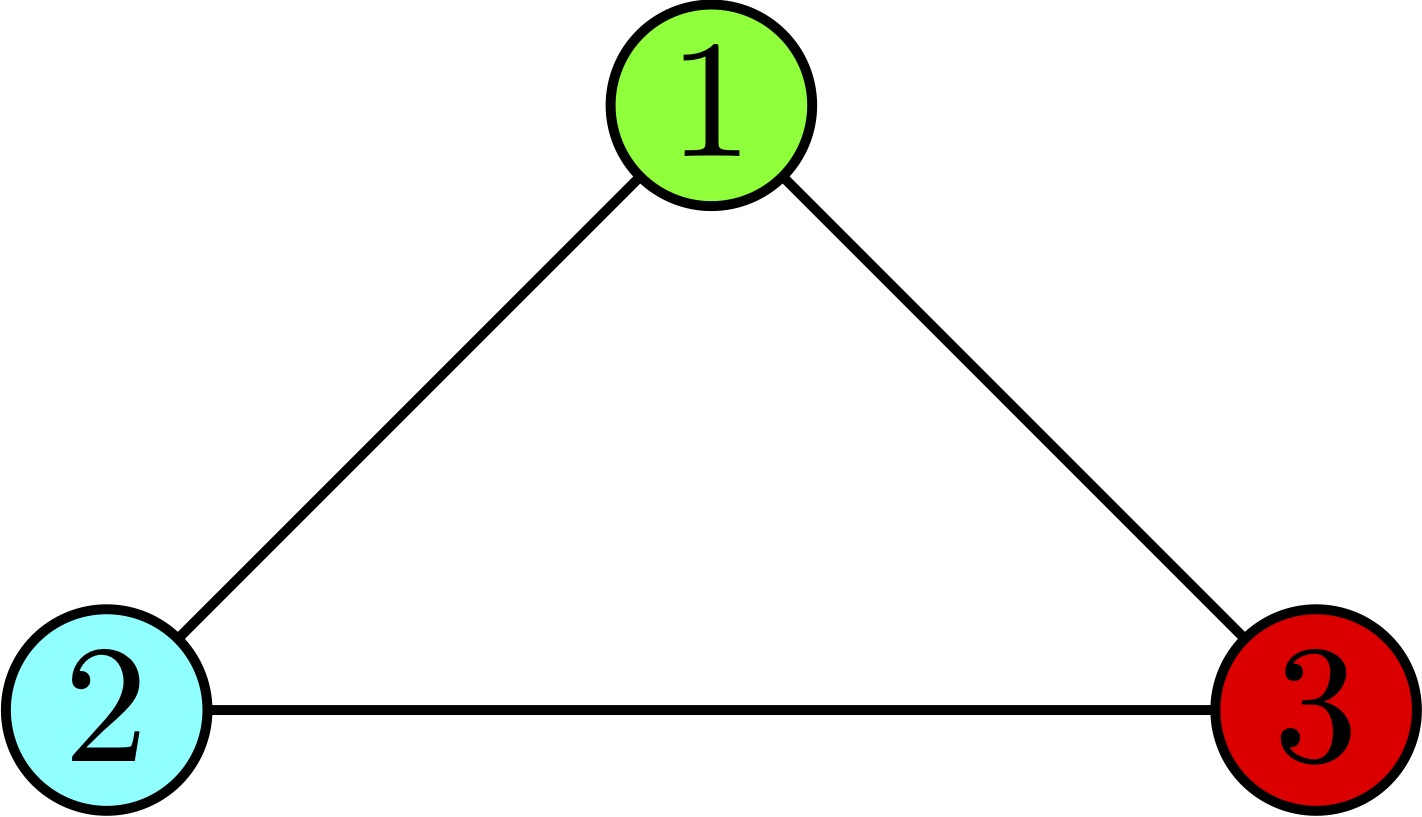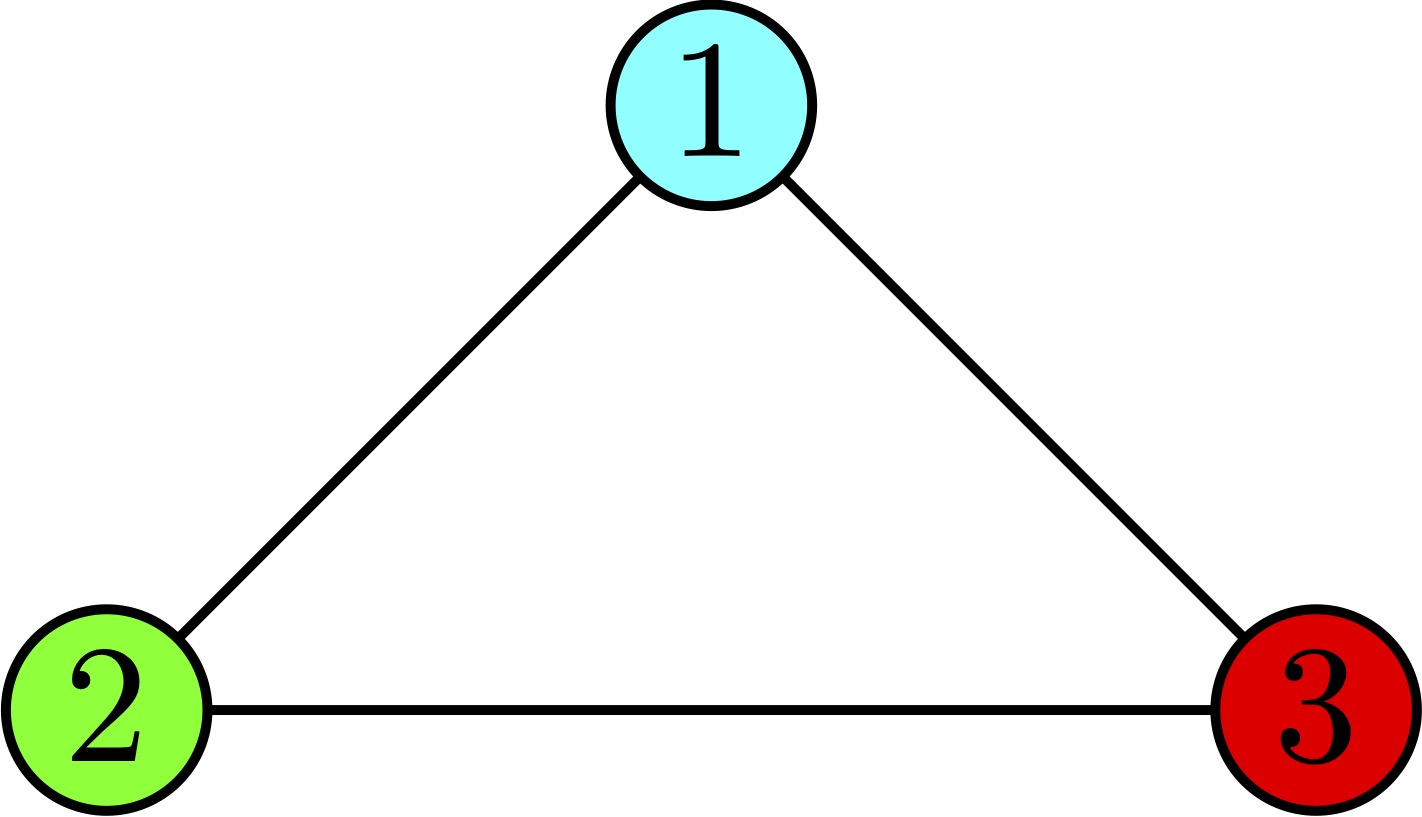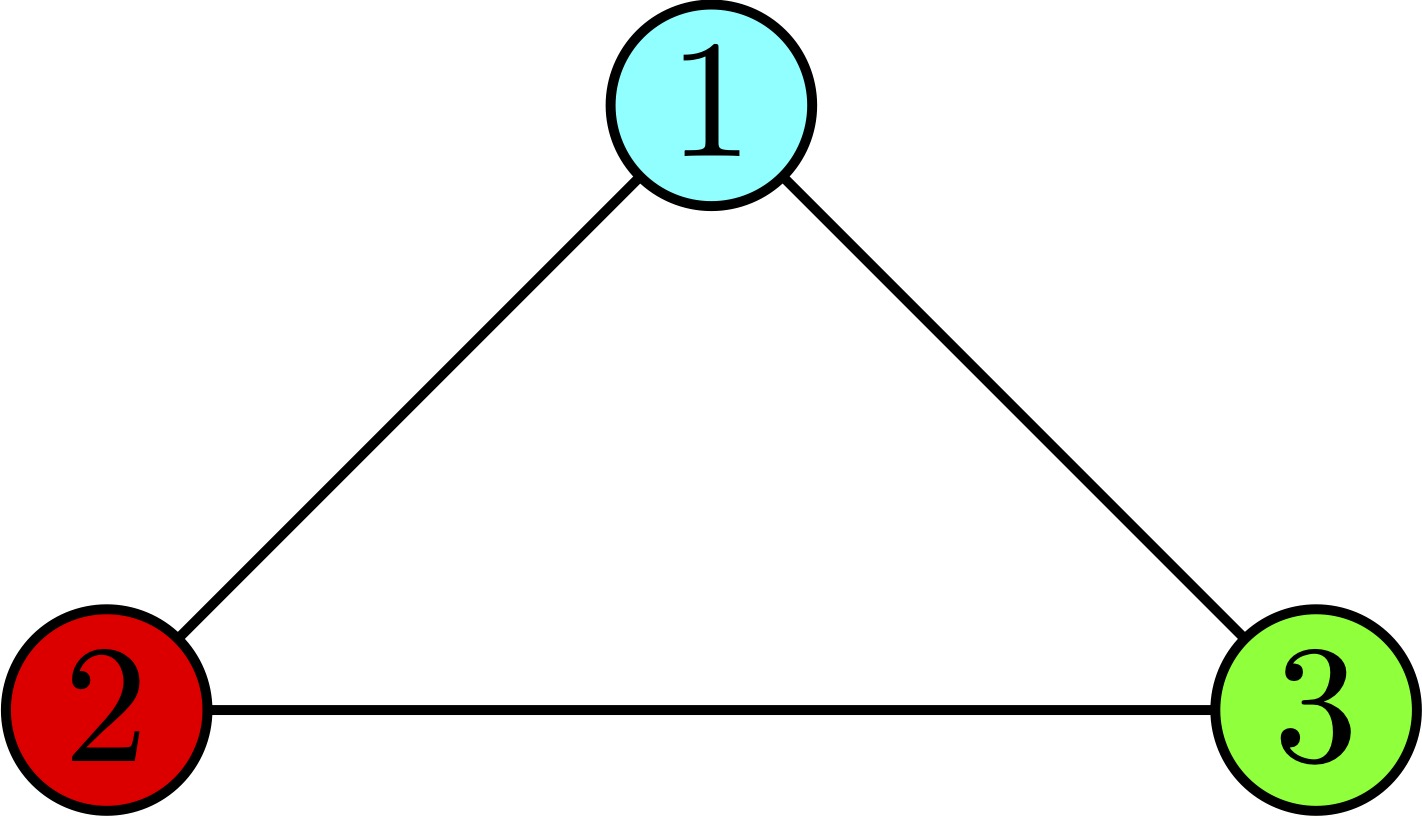시간 제한메모리 제한제출정답맞힌 사람정답 비율
2 초 (추가 시간 없음) 512 MB111100.000%

## 문제

Consider a connected undirected graph on $n$ vertices. Denote this graph as the starting graph.

A tuple of integers $(a, b, c)$~($1 \leq a < b < c \leq n$) is good if and only if

1. Vertices $a$ and $b$ are connected by an edge.
2. Vertices $a$ and $c$ are connected by an edge.
3. Vertices $b$ and $c$ are not connected by an edge.

The following algorithm was applied to the starting graph. While there is one, choose an arbitrary good tuple $(a, b, c)$ and add an edge between vertices $b$ and $c$. Denote the resulting graph as the completed graph. It can be proven that the completed graph does not depend on the choices of good tuple at each iteration.

You are given a starting graph. How many colorings in $n$ colors does the completed graph have? Graph coloring is an assignment of colors to vertices such that no two adjacent vertices share a color. Refer to problem D if you need an even more formal definition.

Output the correct answer modulo 998244353. Formally, if the real answer is $y$ and your answer is $x$, it will be considered correct if $-2^{63} \leq x < 2^{63}$ and $x-y$ is divisible by 998244353.

## 입력

The first line of input contains two integers $n$ and $m$~($2 \leq n \leq 3 \cdot 10^5, 1 \leq m \leq 3 \cdot 10^5$), the number of vertices and edges in the starting graph, respectively.

Next $m$ lines contain descriptions of starting graph edges. The $i$-th of them contains integers $u$ and $v$~($1 \leq u < v \leq n$), the endpoints of the $i$-th edge.

It is guaranteed that the given graph is connected and doesn't contain multiple edges.

## 출력

Print a single integer, the number of colorings in $n$ colors of the completed graph modulo 998244353.

## 예제 입력 1

3 2
1 2
1 3


## 예제 출력 1

6


## 예제 입력 2

8 9
1 2
3 8
1 3
2 6
4 7
5 6
2 5
2 4
7 8


## 예제 출력 2

645120


## 노트

In the first example the starting graph looks like this: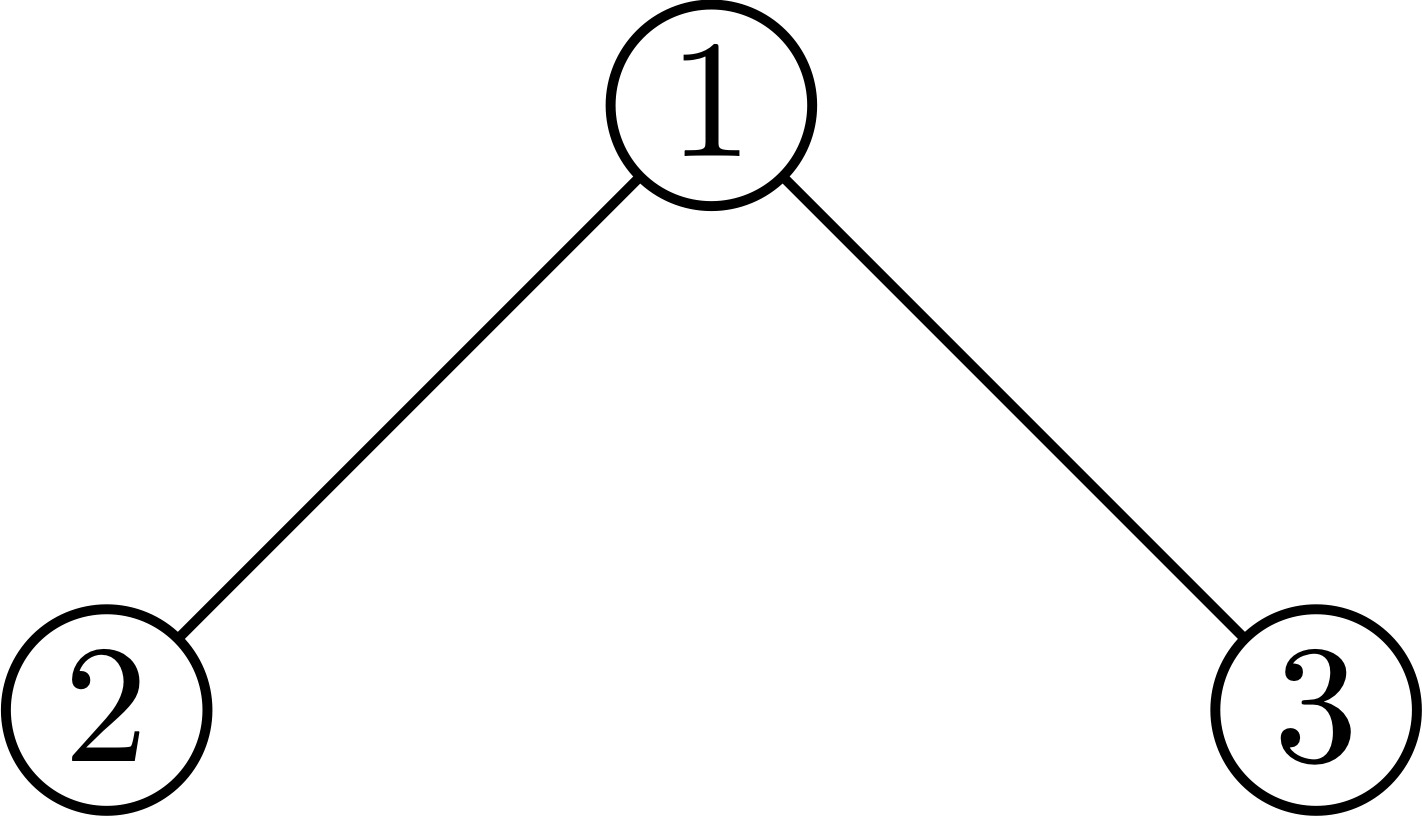The only possible good tuple is $(1, 2, 3)$, so completed graph looks like this: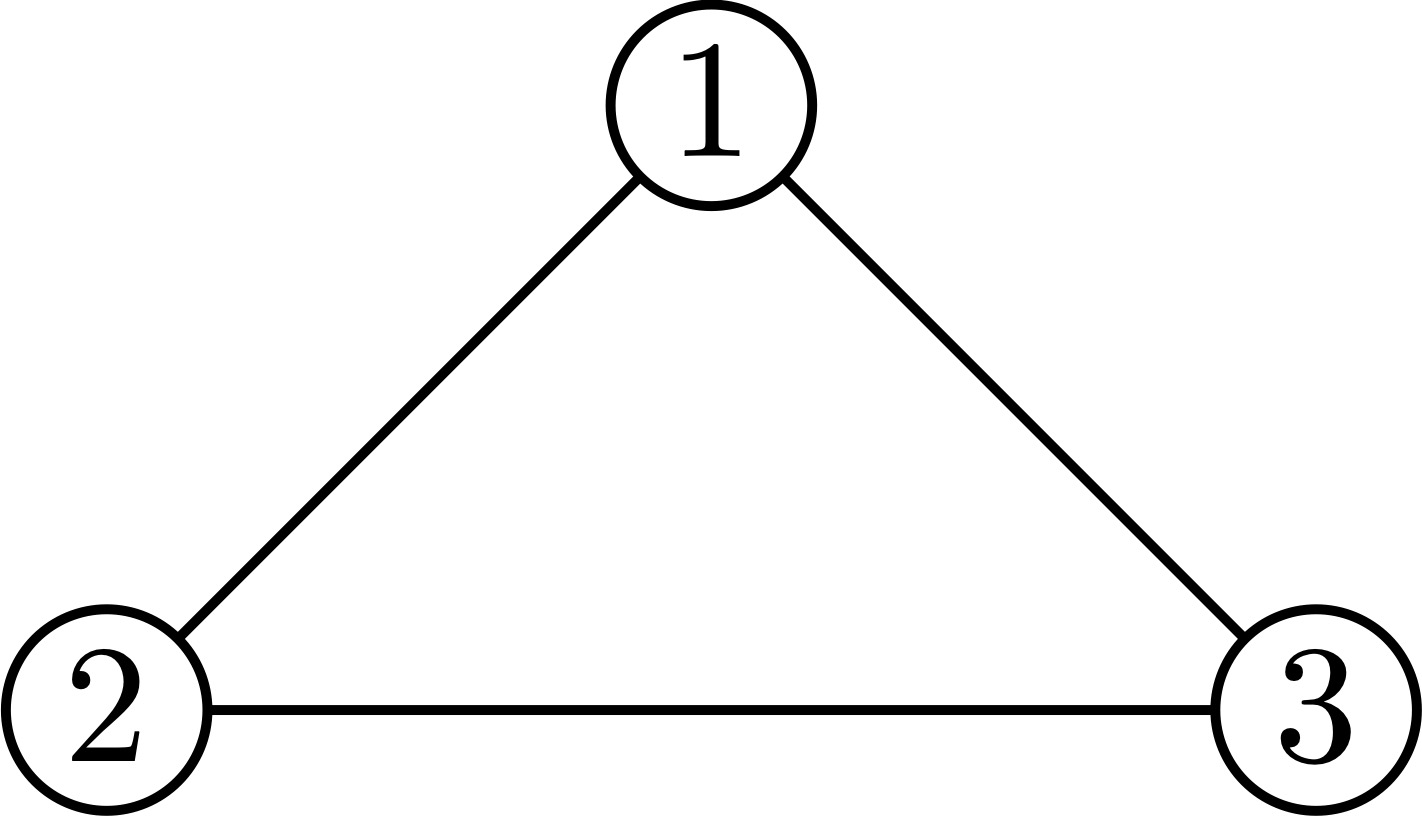Here are all $6$ colorings of such a graph in $3$ colors: